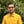Trusted answers to developer questions
Trusted Answers to Developer Questions

Related Tags

swift
communitycreator

# What is the tanh() function in Swift?Harris Amjad

Grokking Modern System Design Interview for Engineers & Managers

Ace your System Design Interview and take your career to the next level. Learn to handle the design of applications like Netflix, Quora, Facebook, Uber, and many more in a 45-min interview. Learn the RESHADED framework for architecting web-scale applications by determining requirements, constraints, and assumptions before diving into a step-by-step design process.

### Overview

The tanh() function in Swift returns a number’s hyperbolic tangent.

Below is the mathematical representation of the tanh() function:

Mathematical representation of the hyperbolic tangent function

Note: We need to import Foundation in our code to use the tanh() function. We can import it like this: import Foundation.

### Syntax

tanh(num)

### Parameter

This function requires a number representing an angle in radians as a parameter.

To convert degrees to radians, use:

radians = degrees * ( pi / 180.0 )

### Return value

This function returns the hyperbolic tangent of a number sent as a parameter.

### Example

The code below shows the use of the tanh() function in Swift:

import Swiftimport Foundation//positive number in radiansprint("The value of tanh(2.3) :", tanh(2.3));// negative number in radiansprint("The value of tanh(-2.3) :", tanh(-2.3));//converting the degrees angle into radians and then applying tanh()// degrees = 45.0// PI = 3.14159265print("The value of tanh(45.0 * (PI / 180.0)) :", tanh(45.0 * (Double.pi / 180.0)));

### Explanation

• Line 2: We add the Foundation header required for tanh() function.
• Line 5: We calculate the hyperbolic tangent of the positive number in radians using tanh().
• Line 8: We calculate the hyperbolic tangent of the negative number in radians using tanh().
• Line 13: We converted the angle in degrees to radians and then calculated its hyperbolic tangent using tanh().

RELATED TAGS

swift
communitycreator

CONTRIBUTORHarris Amjad

Grokking Modern System Design Interview for Engineers & Managers

Ace your System Design Interview and take your career to the next level. Learn to handle the design of applications like Netflix, Quora, Facebook, Uber, and many more in a 45-min interview. Learn the RESHADED framework for architecting web-scale applications by determining requirements, constraints, and assumptions before diving into a step-by-step design process.

Keep Exploring

Learn in-demand tech skills in half the time